# KSEEB Solutions for Class 8 Maths Chapter 13 Direct and Inverse Proportions Ex 13.2

You can Download KSEEB Solutions for Class 8 Maths Chapter 13 Direct and Inverse Proportions Ex 13.2 Questions and Answers helps you to revise the complete syllabus.

## KSEEB Solutions for Class 8 Maths Chapter 13 Direct and Inverse Proportions Ex 13.2

Question 1.
Which of the following are in inverse proportion?
(i) The number of workers on a job and the time to complete the job.
(ii) The time taken for a journey and the distance travelled at a uniform speed.
(iii) Area of cultivated land and the crop harvested.
(iv) The time taken for a fixed journey and the speed of the vehicle.
(v) The population of a country and the area of land per person.
Solution:
(i) Inversely proportional
(ii) Directly proportional
(iii) Inversely proportional
(iv) Inversely proportional
(v) Inversely proportional.Question 2.
In a Television game show, the prize money of Rs 1,00,000 is to be divided equally amongst the winners. Complete the following table and find whether the prize money given to an individual winner is directly or inversely proportional to the number of winners?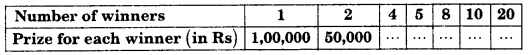Solution:
Since the relation is inversely proportional
∴ Product of number of winners to prize for each winner is constant.
1 × 1,00,000 = 2 × 50,000
4 × x = 1 × 1,00,000
x = $$\frac{1,00,000}{4}$$ = 25,000
y = $$\frac{1,00,000}{5}$$ = 20,000
z = $$\frac{1,00,000}{8}$$ = 12,500
a = $$\frac{1,00,000}{10}$$ = 10,000
b = $$\frac{1,00,000}{20}$$ = 5,000

Question 3.
Rehman is making a wheel using spokes. He wants to fix equal spokes in such a way that the angles between any pair of consecutive spokes are equal. Help him by completing the following table.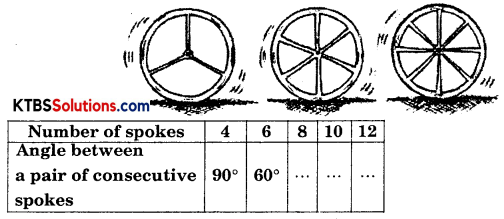(i) Are the number of spokes and the angles formed between the pairs of consecutive spokes in inverse proportion?
(ii) Calculate the angle between a pair of consecutive spokes on a wheel with 15 spokes.
(iii) How many spokes would be needed, if the angle between a pair of consecutive spokes is 40°?
Solution:
Number of spokes to the angle between the pair of consecutive spokes are inversely proportional.
4 × 90° = 6 × 60°
360° = 360°
⇒ 360° = 8 × a
⇒ $$\frac{360^{\circ}}{8}$$ = a
⇒ a = 45°360° = 10 × b
⇒ $$\frac{360^{\circ}}{10}$$ = b
⇒ b = 36°

360° = 12 × c
⇒ $$\frac{360^{\circ}}{12}$$ = c
⇒ c = 30°

(i) Since as the number of spokes increases, the angle between the spokes decreases.
∴ Relation is inversely proportional.

(ii) 4 × 90° = 15 × x (Let angle between spokes = x)
⇒ $$\frac{4 \times 90^{\circ}}{15}$$ = x
⇒ x = 24°
∴ Angle between two consecutive spokes = 24°

(iii) 4 × 90° = 40° × y (Let the number of spokes = y)
⇒ $$\frac{4 \times 90^{\circ}}{40^{\circ}}$$ = y
⇒ y = 9
∴ Number of spokes = 9

Question 4.
If a box of sweets is divided among 24 children, they will get 5 sweets each. How many would each get, if the number of the children is reduced by 4?
Solution: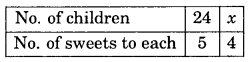Since no. of children and sweets to each are inversely proportional then,
24 × 5 = x × 4
⇒ $$\frac{24 \times 5}{4}$$ = x
⇒ 6 × 5 = x
⇒ x = 30
Hence number of children = 30Question 5.
A farmer has enough food to feed 20 animals in his cattle for 6 days. How long would the food last if there were 10 more animals in his cattle?
Solution: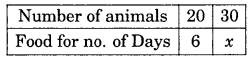Relation between the number of animals and no. of days for food is inversely proportional, then
20 × 6 = 30 × x
⇒ $$\frac{20 \times 6}{30}$$ = x
⇒ x = 4
Hence no. of days = 4

Question 6.
A contractor estimates that 3 persons could rewire Jasminder’s house in 4 days. If, he uses 4 persons instead of three, how long should they take to complete the job?
Solution: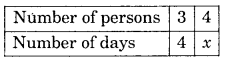The relation between number of persons and number of days varies inversely.
3 × 4 = 4 × x
⇒ $$\frac{3 \times 4}{4}$$ = x
⇒ x = 3
Hence the work will be completed in = 3 days.Question 7.
A batch of bottles were packed in 25 boxes with 12 bottles in each box. If the same batch is packed using 20 bottles in each box, how many boxes would be filled?Solution: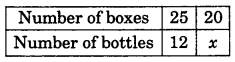Number of boxes and number of bottles varies inversely.
25 × 12 = 20 × x
⇒ $$\frac{25 \times 12}{20}$$ = x
⇒ 5 × 3 = x
⇒ x = 15
∴ Number of boxes will be filled = 15

Question 8.
A factory requires 42 machines to produce a given number of articles in 63 days. How many machines would be required to produce the same number of articles in 54 days.
Solution: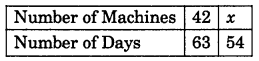Number of machines and number of days varies inversely.
42 × 63 = x × 54
⇒ x = $$\frac{42 \times 63}{54}$$
⇒ x = 49
Hence, the required machines = 49Question 9.
A car takes 2 hours to reach a destination by travelling at the speed of 60 km/h. How long will it take when the car travels at the speed of 80 km/h?
Solution: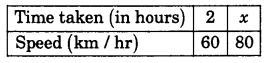Speed and time varies inversely
2 × 60 = x × 80
⇒ $$\frac{2 \times 60}{80}$$ = x
⇒ 1.5 hours = x
Hence, time taken = 1.5 hours.

Question 10.
Two persons could fit new windows in a house in 3 days.
(i) One of the persons fell ill before the work started. How long would the job take now?
(ii) How many persons would be needed to fit the windows in one day?
Solution:
(i)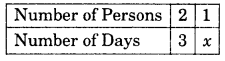No. of persons and number of days varies inversely.
2 × 3 = x × 1
⇒ x = 6
Hence persons will fit a window in 6 days.(ii)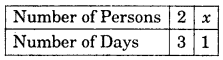2 × 3 = x × 1
⇒ 6 = x
∴ 6 persons will fit the windows in 1 day.

Question 11.
A school has 8 periods a day each of 45 minutes duration. How long would each period be, if the school has 9 periods a day, assuming the number of school hours to be the same?
Solution: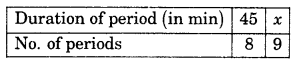Number of periods and duration of period (If total time remain same) varies inversely.
45 × 8 = x × 9
⇒ $$\frac{45 \times 8}{9}$$ = x
⇒ x = 40
Hence if No. of periods are 9, then each period is of duration 40 min.